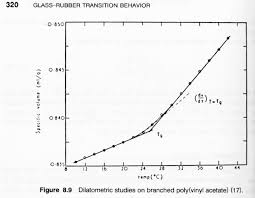## How to Calculate and Solve for Free Volume of Polymer | Polymer & TextileThe image above represents free volume of polymer.

To compute for free volume of polymer, two essential parameters are needed and these parameters are specific volume (V) and volume of solidly packed molecules (Vs).

The formula for calculating free volume of polymer:

Vf = V – Vs

Where:

Vf = Free Volume of Polymers
V = Specific Volume
Vs = Volume of Solidly Packed Molecules

Let’s solve an example;
Find the free volume of polymer when the specific volume is 30 and the volume of solidly packed molecules is 10.

This implies that;

V = Specific Volume = 30
Vs = Volume of Solidly Packed Molecules = 10

Vf = V – Vs
Vf = 30 – 10
Vf = 20

Therefore, the free volume of the polymer is 20.

Calculating the Specific Volume when the Free Volume of Polymer and the Volume of Solidly Packed Molecules.

V = Vf + Vs

Where;

V = Specific Volume
Vf = Free Volume of Polymers
Vs = Volume of Solidly Packed Molecules

Let’s solve an example;
Find the specific volume when the free volume of polymer is 24 and the volume of solidly packed molecules is 12.

This implies that;

Vf = Free Volume of Polymers = 24
Vs = Volume of Solidly Packed Molecules = 12

V = Vf + Vs
V = 24 + 12
V = 36

Therefore, the specific volume is 36.